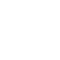# Steady Flow Energy Equation (SFEE)

Consider an open system through in which the working substance flows as a steady rate as shown in figure. The working substance entering the system at (1) and leaving the system at (2).

Let, p1 be the pressure of the working substance entering the system (N/m²)

v1 be the specific volume of the working substance entering the system in m³/kg.

C1 be the velocity of the working substance entering the system

u1 be the specific internal energy of the working substance entering the system in J/kg.

z1 be the height above the datum level for inlet in m

p2,v2,C2, u2 and z2 are corresponding values for the working substance leaving the system.

Q be the heat supplied to the system in J/kg

W be the work delivered by the system in J/kg

∴ Total energy entering the system,

$\begin{array}{l} =P \cdot E+K \cdot E+I \cdot E+F \cdot E+\text { Heat energy } \\ =g z_{1}+\frac{C_{1}^{2}}{2}+u_{1}+p_{1} v_{1}+Q \end{array}$

Total energy leaving the system,

$=P . E+K . E+I E+F \cdot E+\text { Work }$ $=g z_{2}+\frac{C_{2}^{2}}{2}+u_{2}+p_{2} v_{2}+W$

By first law of thermodynamics,

Total energy entering the system = Total energy leaving the system

$\begin{array}{c} g z_{1}+\frac{C_{1}^{2}}{2}+u_{1}+p_{1} v_{1}+Q=g z_{2}+\frac{C_{2}^{2}}{2}+u_{2}+p_{2} v_{2}+W \\ {[\because h=u+p v]} \\ \qquad g z_{1}+\frac{C_{1}^{2}}{2}+h_{1}+Q=g z_{2}+\frac{C_{2}^{2}}{2}+h_{2}+W \end{array}$

The above equation is known as steady flow energy equation (SFEE). The above equation represents the energy flow per unit of mass of the working substance (J/kg). When the equation is multiplied by mass of the working substance throughout, then all terms will represent the energy flow per unit time. (in J/s) Then the above equation becomes.

$m\left(g z_{1}+\frac{C_{1}^{2}}{2}+h_{1}+Q\right)=m\left(g z_{2}+\frac{C_{2}^{2}}{2}+h_{2}+W\right)$

If the values of Q and W in kJ/kg, and h1 and h2 are substituted in kJ/kg, then the above equation becomes,

$m\left(\frac{g z_{1}}{1000}+\frac{C_{1}^{2}}{2000}+h_{1}+Q\right)=m\left(\frac{g z_{2}}{1000}+\frac{C_{2}^{2}}{2000}+h_{2}+W\right)$

If Q and W are already kW, and h1 and h2 are substituted in kJ/kg, then the above equation becomes,

$m\left(\frac{g z_{1}}{1000}+\frac{C_{1}^{2}}{2000}+h_{1}\right)+Q=m\left(\frac{g z_{2}}{1000}+\frac{C_{2}^{2}}{2000}+h_{2}\right)+W$

Note : In a steady flow system, the mass rate of the working substance is given by

$m=\frac{A_{1} C_{1}}{v_{1}}=\frac{A_{2} C_{2}}{v_{2}}$

where,

The mass is represented in kg/s.
A1 and A2 are areas of cross section at entry and exit in .